# Physics - Classical Mechanics - Damped Oscillation

in STEMGeeks2 years ago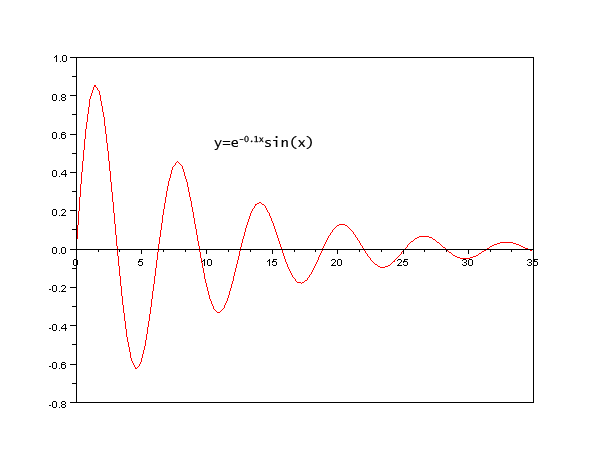[Image1]

## Introduction

Hey it's a me again @drifter1!

Today we continue with Physics, and more specifically the branch of "Classical Mechanics" in order to get into Damped Oscillation.

So, without further ado, let's get straight into it!

## Damped Oscillation

The period motions that we've covered up to this point were all ideal, with no friction. In real systems, there is always a force that "damps" down the oscillator, eventually stopping the oscillation completely.

### Damping Force

These damping forces can become quite complicated. The simplest one which is kinetic friction only depends on the velocity, so let's use this one for our model.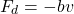In this equation b is the damping constant or coefficient, which specifies the strength of the damping.

### Total Force and Differential Equation

Adding this force to the simple harmonic motion model, yields: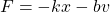Of course, from Newton's 2nd Law this also equals ma. Let's also introduce derivatives of x. This gives us the following quadratic differential equation: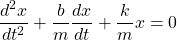### Motion Equations

The solution to the previous differential equation is known. It's an oscillating cosine in the envelope of an exponential function, an exponentially decaying sinusoidal. Thus, the displacement in a damping oscillation is given by: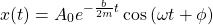The corresponding angular frequency (ω) is: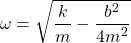Substituting SHM's angular frequency, which is referred to as the natural angular frequency (ω0), yields: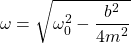### Special Cases

Depending on the value of b, there are three cases for an Damping Oscillator: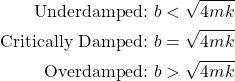As b increases ω eventually reaches zero. If it increases even further it becomes a complex number.

In an underdamped system the amplitude decreases exponentially, eventually putting the system to rest. When equal to zero (critical damping), the system doesn't oscillate at all. And lastly, an overdamped system takes much longer to reach equilibrium than the other two and also doesn't oscillate.

More specifically, for the last case, the solution can also be written as: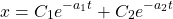This is the same behavior as electronic circuits!

## Final words | Next up

And this is actually it for today's post!

Next time we will get into Forced Oscillation and Resonance...

See ya!Keep on drifting!

Posted with STEMGeeks

Sort:
2 years ago Reveal Comment